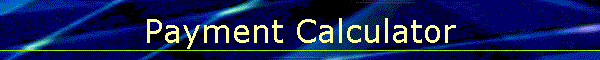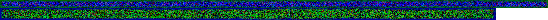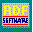Calculates for any one unknown: Payment, Rate, Term in years, or Principal Amount for simple 'Average Balance' based interest loans.   Need Instructions ??

Loan Name:
Principal Amount:    *  1st Pmt Month#
Interest Rate:   *       Payments: *
Periods Per year:       Length in Years: *

* = 4 parameters one unknownSend mail to RDFoerster@msn.com with questions or comments about this web site.
Copyright © 1998 RDFoerster Software
Last modified: June 04, 2006

Calculation Information:

Use of Internet Explorer is Required!

You should always check with the lender to determine exactly how the interest is calculated for a specific loan.  The Calculations used here to determine payment and number of payments are fairly commonly used, and should provide a reasonably close calculation of key data for most common consumer loans.  The calculations to determine the interest rate and principal amount produce 'estimates' only.  You can plug these back in and recalculate the loan to determine the actual deviation.  With a few adjustments you should be able to arrive at a close result.

Payment calculation
is based on the Formula: P=A*R/1-(1+R)^-N)    where R is the
monthly Interest Rate equivalent. P=Payment, A=Principal, N=Number of Payments.

Number of Payments Calculation
is based on the Formula: N =-(LOG(1-A*R/P))/LOG(1+R) where R is the monthly Interest Rate equivalent. P=Payment, A=Principal, N=Number of Payments.

Rate Estimate Calculation
is based on the Formula: Rte =(2/Y)-(2/(N*P*Y)* A where Rte is the Annual Interest Rate, P=Payment, A=Principal, N=Number of Payments, and Y= Length of loan in years.
To improve this estimate, successive approximation is then used to calculate the payment while adjusting the rate until the payment closely matches the adjusted rate.

Principal Amount Calculation is based on the Formula:
A = (P/Rte)*Prd*(1-(1 + Rte/Prd)^-(YR/Prd))
where Rte is the Annual Interest Rate, P=Payment, A=Principal, N=Number of Payments, and Prd=Number of Payments per year. To improve this estimate, successive approximation is then used to calculate the payment while adjusting the Principal Amount until the payment closely matches the adjusted Principal Amount.